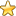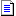The Main Number Sections:
0 - 500 | 501 - 1000 | 1001 - 1500 | 1501 - 2000 | 2001 - 2500
Number Articles | Higher Numbers | Random Number
You are here: HomeThe Database of Number Correlations# The Chemical Elements

This page is organized according to the theories of Peter Plichta as set out in his book "God's Secret Formula". For a more conventional arrangement see the award winning
Web Elements website.

There are 81 stable Chemical Elements.

 Potassium(19)
 Beryllium(4) Helium(2) Carbon(6) Lithium(3)

 Single Isotope (atomic numbers are odd) Double Isotopes (atomic numbers are odd) Multiple Isotopes (atomic number divisible by 4) Multiple Isotopes (atomic number divisible by 2) Fluorine(9) Hydrogen(1) Oxygen(8) Neon(10) Phosphorus(15) Boron(5) Magnesium(12) Silicon(14) Scandium(21) Nitrogen(7) Nickel(28) Argon(18) Manganese(25) Chlorine(17) Germanium(32) Titanium(22) Cobalt(27) Vanadium(23) Zirconium(40) Zinc(30) Arsenic(33) Copper(29) Ruthenium(44) Tungsten(74) Yttrium(39) Gallium(31) Barium(56) Molybdenum(42) Rhodium(45) Rubidium(37) Neodymium(60) Samarium(62) Caesium(55) Silver(47) Gadolinium(64) Dysprosium(66) Terbium(65) Lutetium(71) Osmium(76) Ytterbium(70) Thulium(69) Tantalum(73) Mercury(80) Xenon(54) Sodium(11) Bromine(35) Sulfur(16) Iron(26) Aluminum(13) Indium(49) Chromium(24) Strontium(38) Niobium(41) Antimony(51) Calcium(20) Cerium(58) Iodine(53) Lanthanum(57) Krypton(36) Lead(82) Praseodymium(59) Europium(63) Erbium(68) Selenium(34) Holmium(67) Rhenium(75) Hafnium(72) Palladium(46) Gold(79) Iridium(77) Cadmium(48) Platinum(78) Bismuth(83) Thallium(81) Tellurium(52) Tin(50)

The table above shows an amazing mathematical order. It divides the building blocks of Nature into 4 x19 chemical elements, where four elements (Beryllium, Helium, Carbon and Lithium), are set above in a super-order. Over them all, sits outstanding, the key-number of this whole system, the number 19, which represents Potassium . The whole system is structured on the number 19.

Of the 81 stable elements:
The total number of pure isotopes is exactly 20.

The total number of double isotopes is exactly 20.

The total number of multiple isotopes divisible by 2 is 20.

The total number of multiple isotopes divisible by 4 is 20.

The largest Neutron number for any stable isotope=209=11x19

From unstable element 43 to unstable element 61 inclusively we have 19 elements.

The total neutron number for all the stable isotopes=30704=1616x19

The total electron number for all the stable elements=1+2+3...83-(43+61)=3382=178x19

Therefore the total proton number for all the stable elements also=1+2+3...83-(43+61)=3382=178x19

The addition of the above 3 totals=37468=19x1972

This external link also gives a description of the number 19 structure intergral to a deeper understanding of the Chemical Elements:
Submission.org..

You are here: HomeThe Database of Number CorrelationsThe Main Number Sections:
0 - 500 | 501 - 1000 | 1001 - 1500 | 1501 - 2000 | 2001 - 2500
Number Articles | Higher Numbers | Random Number